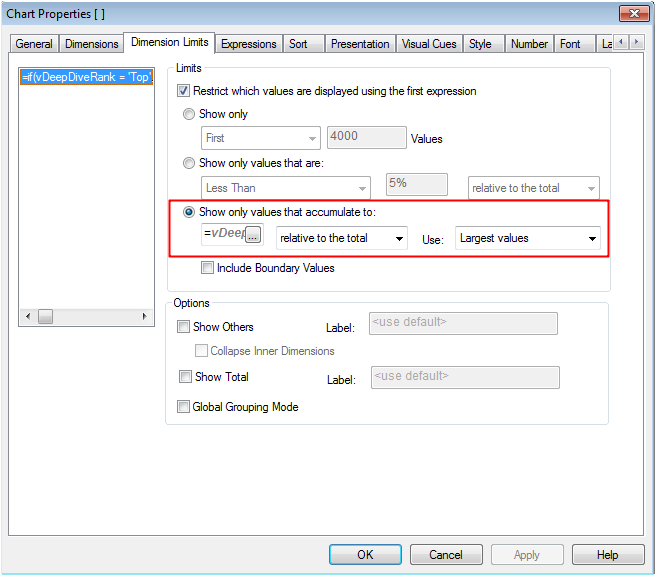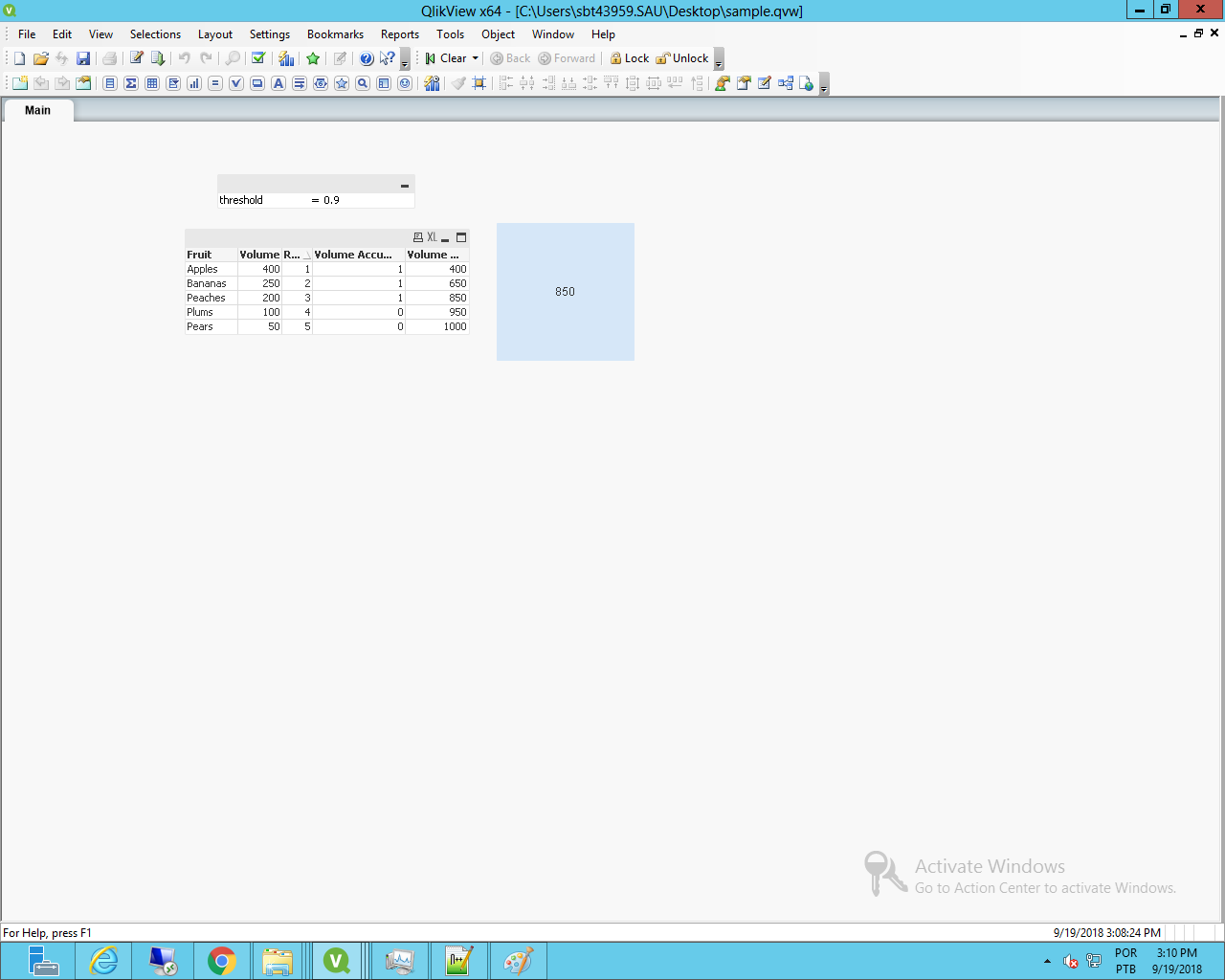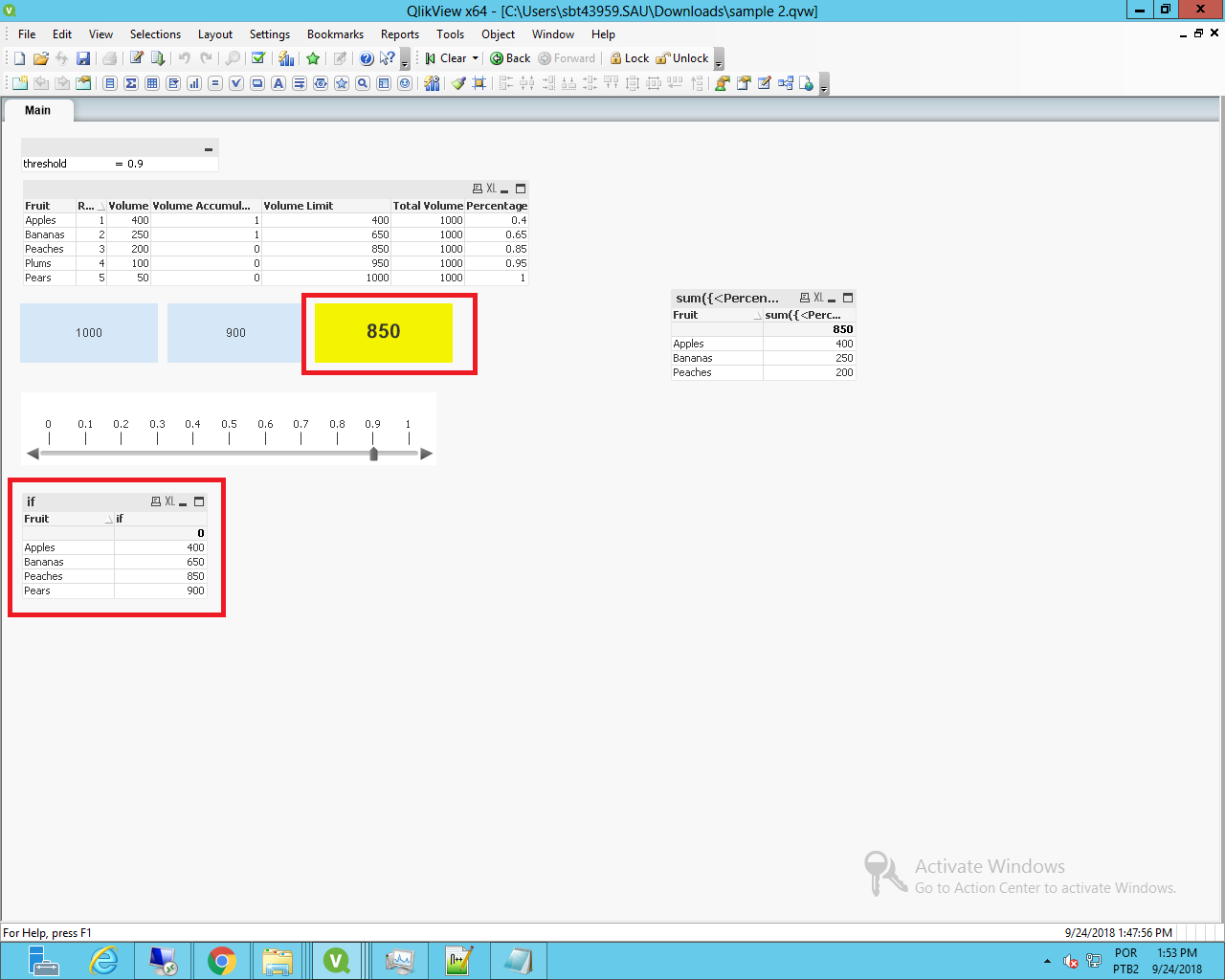# QlikView App Development

Discussion Board for collaboration related to QlikView App Development.

Announcements
Save the Date: QlikWorld Online, June 24-25, 2020. Free global virtual event for data integration and data analytic gurus. Register Today
Highlighted
New Contributor

## Sum if accumulated value is n% of Total

Hi, I have one field [Volume] and I would like to sum rows only if them, once sorted from biggest to smallest, are n% of Total [Volume].

I found this function in chart and it works perfectly.How can I get it Text Expression?

Suppose I have:

FruitVolumeRank
Bananas2502
Apples4001
Pears505
Peaches2003
Plums1004

Total [Volume] = 1000

I would like to sum only top values within 70% of Total Volume.

Expected result should be:

650

(Apples, 400 + Bananas 250, the first / top 2 values that if summed are <= 70% of Total)

1 Solution

Accepted Solutions
HighlightedPartner

## Re: Sum if accumulated value is n% of Total

Hi,

Attached a solution with a variable table that gets what you need Clemente.

Theres a slider on the right side that lets you see which values are greater than the total volume value multiplied by the threshold.

7 Replies
HighlightedPartner

## Re: Sum if accumulated value is n% of Total

Hi Clemente,

You could do something like the code below:

```//let threshold = 0.7;

data:
[
Fruit,Volume,Rank
Bananas,250,2
Apples,400,1
Pears,50,5
Peaches,200,3
Plums,100,4
];

// get the sum of all, as to get the total vloume
sum:
sum(Volume) as [Total Volume]
Resident data;

// creates variable to get the total amount
let limitVolume = peek('Total Volume',0,'sum');

drop table sum;

// orders the data based on rank and volume values and checks if the aggregated sum is greater than the threshold
NoConcatenate
orderedData:
if([Volume Limit]>\$(limitVolume)*\$(threshold),0,1) as [Volume Accumulate Threshold];
Alt(peek([Volume Limit]),0)+Volume as [Volume Limit]

Resident data
order by Rank,Volume;

drop table data;

```

As expression, simply use the [Volume Accumulate Threshold] field in the set analysis, getting the following:You can change the threshold variable to the limit you want, and get the according values, I've put to 0.9 (90% of limit) and got the sum of the corresponding values.

Attached the QVW used.

Highlighted
New Contributor

## Re: Sum if accumulated value is n% of Total

Thank you so much for your reply Felip. I understood logic behind, brilliant. But what about having it in a textbox formula? I mean, it should be great if you could have threshold selected by user and dynamically formula applied. Without re-launching set analysis.

SUM({<Fruit={"=max(aggr(sum(Volume),Fruit),5))/sum(Total Volume)<=\$(=Threshold)"}>}Volume)

Could you please validate / correct?

Thank you.

HighlightedPartner

## Re: Sum if accumulated value is n% of Total

Hi,

Attached a solution with a variable table that gets what you need Clemente.

Theres a slider on the right side that lets you see which values are greater than the total volume value multiplied by the threshold.

Highlighted
New Contributor

## Re: Sum if accumulated value is n% of Total

Hi Felip, thank you again, we are closer and closer. I used your helpful sample file to create a text box (in yellow, see also attached file + application). The goal is to have textbox with number. Slider is wonderful!

Thank you.

ClementeHighlighted
New Contributor

## Re: Sum if accumulated value is n% of Total

Here it is application I forgot. Sorry.

HighlightedPartner

## Re: Sum if accumulated value is n% of Total

Problem with using the KPI format is that the above function wont work, since there's nothing to check above the current line being calculated.

That's whats done on the simple graph below, it sums up the above value, when on the "Bananas" row, and sums up the current row volume, getting the 400 of apple volume and 250 of bananas.

HighlightedPartner

## Re: Sum if accumulated value is n% of Total

Hi Clemente,

See the attached solution for it.

Keep in mind that it necessarily needs a ranking position, based on the volume or any other metric, since its ordered that way to get the "Percentage" field.